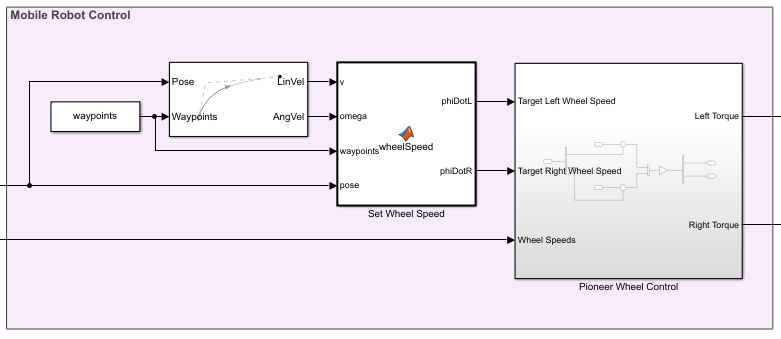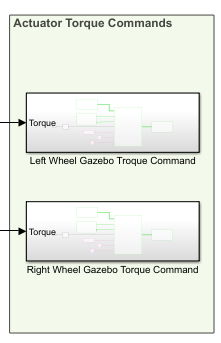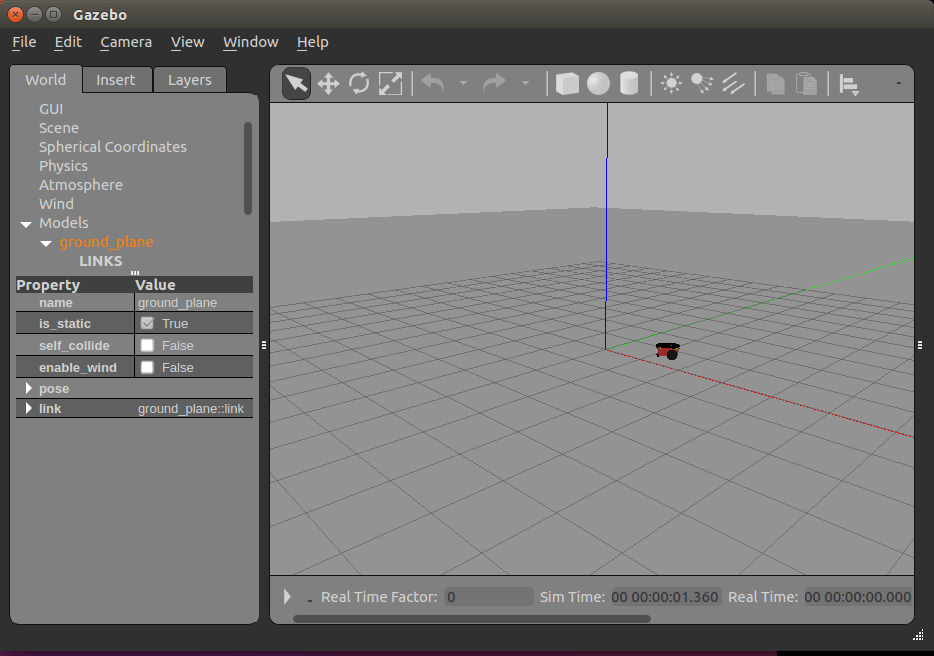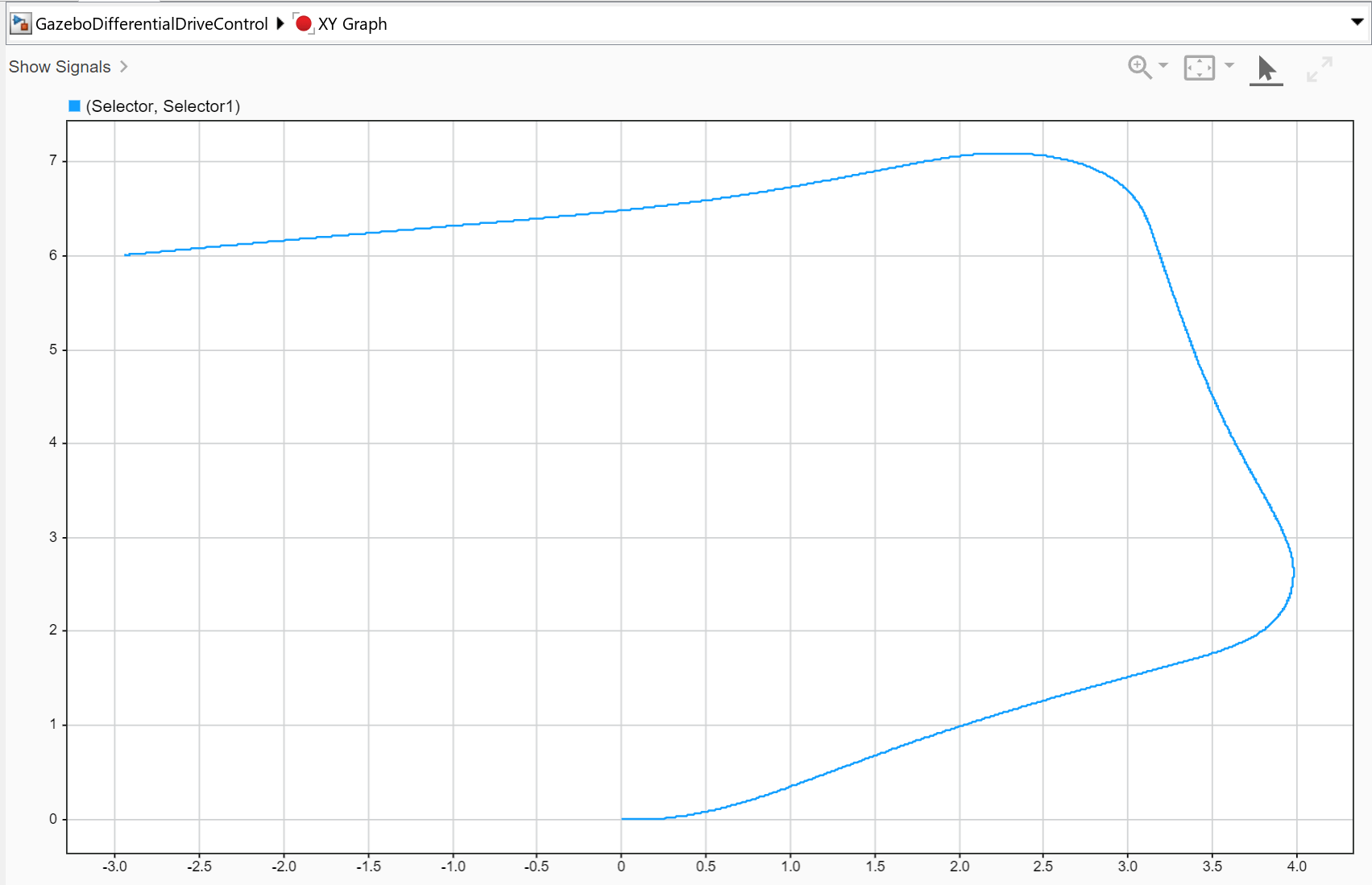# Control A Differential-Drive Robot in Gazebo With Simulink

This example shows how to control a differential drive robot in Gazebo co-simulation using Simulink. The robot follows a set of waypoints by reading the pose and wheel encoder positions and generates torque-control commands to drive it.

To download the virtual machine (VM) used in this example, see Virtual Machine with ROS 2 Bouncy and Gazebo.

For an introduction to Gazebo co-simulation and getting connected for the first time, see Perform Co-Simulation between Simulink and Gazebo.

#### Run the VM

Open the virtual machine installed from Virtual Machine with ROS 2 Bouncy and Gazebo.

### Gazebo World

This example uses a world given in the VM, `differentialDriveRobot.world`, as a simple ground plane with default physics settings. The world uses a Pioneer robot with the default controllers removed, so that the built-in controllers do not compete with torques provided from Simulink. The Pioneer robot is available in default Gazebo installs. The Gazebo plugin references the plugin required for the connection to Simulink, as detailed in Perform Co-Simulation between Simulink and Gazebo.

Double-click the Gazebo Differential Drive Robot icon.

Alternative, run these commands in the terminal:

```cd /home/user/src/GazeboPlugin/export export SVGA_VGPU10=0 gazebo ../world/differentialDriveRobot.world ```### Model Overview

Open the model:

`open_system('GazeboDifferentialDriveControl') `

The model has four sections:

• Gazebo Pacer

• Control Mobile Robot

• Send Actuation Data to Gazebo

#### Gazebo PacerThis section establishes the connection to Gazebo. Double-click the Gazebo Pacer block to open its parameters, and then click the Configure Gazebo network and simulation settings link. This will open a dialog.

Specify the IP Address for your VM. By default, Gazebo connects on the `14581` port. Click the Test button to verify the connection to Gazebo.If the test is not successful, make sure to check the instructions in Perform Co-Simulation between Simulink and Gazebo and ensure that Gazebo is properly configured and the associated world is up and running.

#### Gazebo Sensor Outputs

The sensor outputs read sensor data from Gazebo and passes it to the appropriate Simulink blocks. An XY graph plots the current robot position, and pose data is saved to the simulation output.The Read Gazebo Sensors subsystem extracts the robot pose and wheel sensor data. The pose data are the xy-coordinates and a four-element quaternion for orientation. The wheel speeds are computed based on rate of change of the wheel positions as they rotate.#### Mobile Robot Control

The Mobile Robot Control section accepts a set of target waypoints, current pose, and the current wheel speeds, and outputs the wheel torques needed to have the robot follow a path that pursues the waypoints.There are three main components.

The Pure Pursuit block is a controller that specifies the vehicle speed and heading angular velocity of the vehicle needed to follow the waypoints at a fixed speed, given the current pose.

The Set Wheel Speed MATLAB Function block converts vehicle speed and heading angular velocity to left and right wheel speed, using the kinematics of a differential-drive robot:

`${\stackrel{˙}{\varphi }}_{\mathit{L}}=\frac{1}{\mathit{r}}\left(\mathit{v}-\frac{\mathrm{𝜔d}}{2}\right)\text{\hspace{0.17em}}$`

`${\stackrel{˙}{\varphi }}_{\mathit{R}}=\frac{1}{\mathit{r}}\left(\mathit{v}+\frac{\mathrm{𝜔d}}{2}\right)\text{\hspace{0.17em}}\text{\hspace{0.17em}}$`

${\stackrel{˙}{\varphi }}_{\mathit{L}}$ and ${\stackrel{˙}{\varphi }}_{\mathit{R}}$ are the left and right wheel speeds, $\mathit{v}$ is the vehicle speed, $𝜔$ is the vehicle heading angular velocity, $\mathit{d}$ is the track width, and $\mathit{r}$ is the wheel radius. Additionally, this MATLAB® Function includes code to throttle the wheel speed. Since the Pure Pursuit block uses a fixed speed throughout, inside the MATLAB Function block, there are two if-statements. The first slows the velocity at a rate proportional to the distance to the goal when the robot is within a certain distance threshold. The second if-statement stops the robot when it is within a tight threshold. This helps the robot to come to a gentle stop.

Finally, the Pioneer Wheel Control subsystem converts the desired wheel speeds to torques using a proportional controller.

#### Actuator Torque Commands

The last section of the model takes the torque commands produced by the controller and sends it to Simulink using blocks from the Gazebo Co-Simulation Library.Inside each of the subsystems in this block, a Bus Assignment block is used to assign the joint torque to the correct joint.For example, inside the Left Wheel Gazebo Torque Command subsystem, shown above, a Gazebo Blank Message with the `ApplyJointTorque` command type is used to specify the bus type. The model and joint name are provided by the Gazebo Select Entity block, which is linked to the joint associated with the left wheel in the Gazebo world, `left_wheel_hinge`. The torque is applied for the entire step time, 0.01 seconds, specified in nanoseconds since these inputs must be provided as integers. The output of the bus is passed to a Gazebo Apply Command block.

### Simulate the robot

To run the model, initialize the waypoints and set the sample time:

```waypoints = [0 0; 4 2; 3 7; -3 6]; sampleTime = 0.01;```

Click Play button or use the `sim` command to run the model. During execution, the robot should move in Gazebo, and an XY Plot updates the pose observed in Simulink.The figures plot the set of waypoints and the final executed path of the robot.